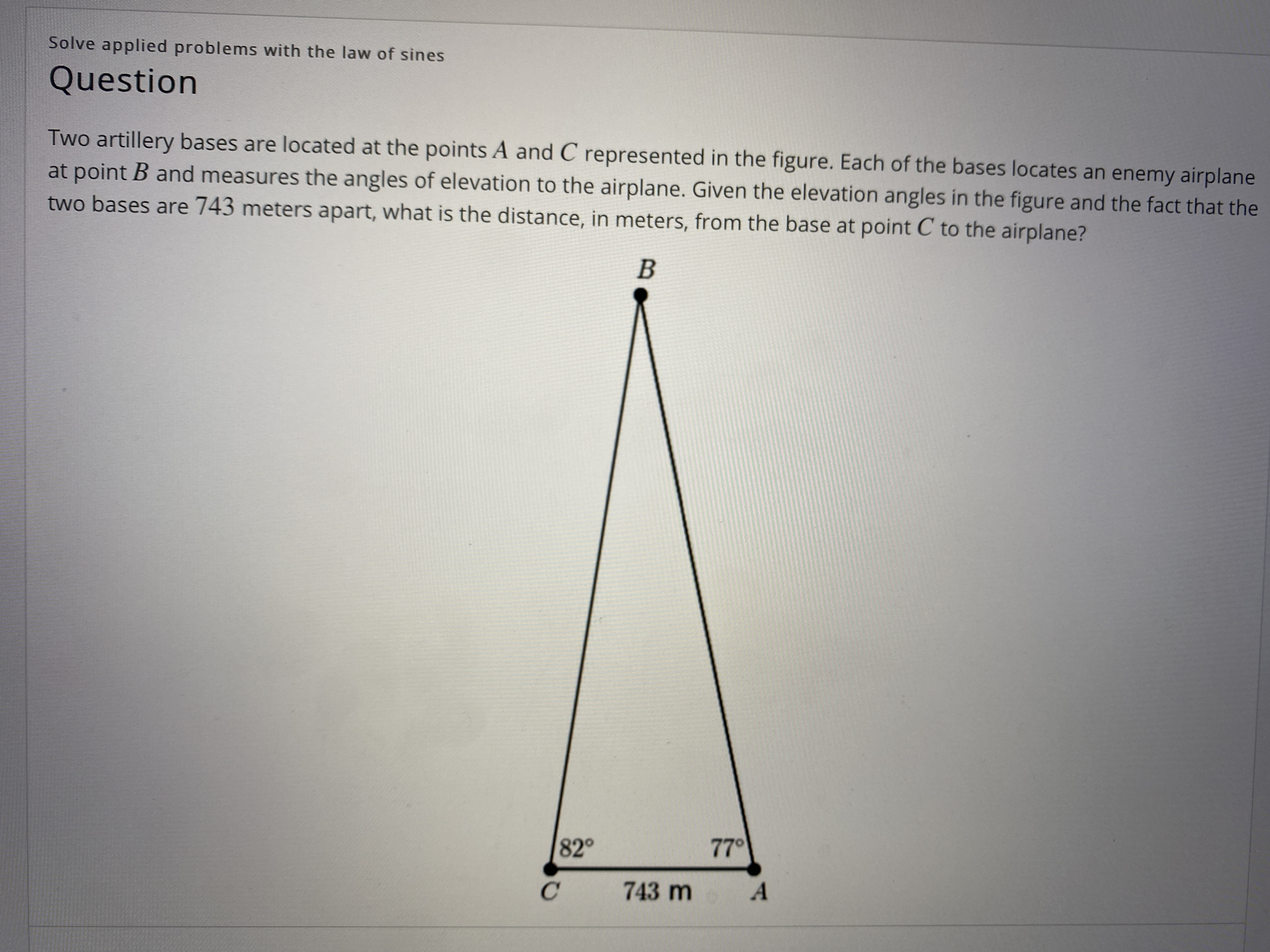# Two artillery bases are located at the points A and C represented in the figure. Each of the bases locates an enemy airplane at point B and measures the angles of elevation to the airplane. Given the elevation angles in the figure and the fact that the two bases are 743 meters apart, what is the distance, in meters, from the base at point C to the airplane? B

Questionhelp_outlineImage TranscriptioncloseTwo artillery bases are located at the points A and C represented in the figure. Each of the bases locates an enemy airplane at point B and measures the angles of elevation to the airplane. Given the elevation angles in the figure and the fact that the two bases are 743 meters apart, what is the distance, in meters, from the base at point C to the airplane? B fullscreen

### Want to see this answer and more?

Experts are waiting 24/7 to provide step-by-step solutions in as fast as 30 minutes!*

*Response times may vary by subject and question complexity. Median response time is 34 minutes for paid subscribers and may be longer for promotional offers.
Tagged in
Math
Trigonometry

### Trigonometric Ratios